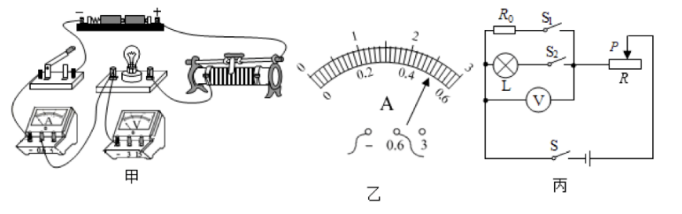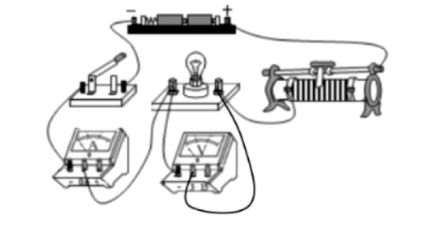(1) 如图甲所示为该小组一同学所连的实验电路, 闭合开关前发现电路中有一根导线末连接, 请把电 路连接完整。
（2）当闭合开关时, 发现电流表无示数, 灯泡不亮, 电压表示数接近电源电压。造成这一现象的原因 可能是小灯泡
（3）排除电路故障后闭合开关, 移动滑片, 当灯泡正常发光时, 电流表示数如图乙所示, 则灯泡的额 定功率为 W。
(4) 同组的小红认为, 可以利用图甲的装置多次测量不同电压下灯泡的电阻, 并求出平均值, 小明认 为小红的观点不合理, 理由是
(5) 小红在继续实验时发现电流表突然损坏, 于是又找来了一个阻值为 $R_0$ 的定值电阻, 设计了如图丙 所示的电路, 已知电源电压为 $\mathrm{U}$ 且保持不变, 也可以测出另一额定电压为 $\mathrm{U}$ 的小的泡的额定功率, 请 将步骤补充完整:
(1)只闭合 $S 、 S_2$, 移动滑片 $P$, 使电压表的示数为
(2)只闭合 ，保持滑片 $\mathrm{P}$ 不动, 读出电压表的示数为 $\mathrm{U}_1$;
(3)小灯泡的额定功率 $\mathrm{P}=$ （用 $U 、 U_1 、 U_{\text {頪、 }} R_0$ 表示）。（2）电流表无示数, 灯泡不亮, 说明电路是断路, 电压表示数接近电源电压, 说明与电压表并联部分 以处的电路是通路, 故造成这一现象的原因可能是小灯泡断路;

(3) 当小灯泡正常工作时, 灯泡两端的电压为额定电压 $2.5 \mathrm{~V}$, 如图乙所示, 电流表选用的是小量程, 分度值为 $0.02 \mathrm{~A}$, 电流表的示数为 $0.5 \mathrm{~A}$, 根据功率公式可得小灯泡的额定功率为: $\mathrm{P}=\mathrm{UI}=2.5 \mathrm{~V} \times 0.5 \mathrm{~A}$ $=1.25 \mathrm{~W}$

（3）利用图甲的装置多次测量不同电压下灯泡的电阻, 并求出平均值, 小明认为小红的观点不合理, 理由是小灯泡的电阻随温度的升高而增大, 不是一个定值, 求平均值没有意义;

(4) ①只闭合 $\mathrm{S} 、 \mathrm{~S}_2$, 移动滑片 $\mathrm{P}$, 使电压表的示数为 $\mathrm{U}$ 额;
②只闭合 $S 、 S_1$, 保持滑片 $P$ 不动, 读出电压表的示数为 $U_1$;

③只闭合 $\mathrm{S} 、 \mathrm{~S}_1, \mathrm{R}_0$ 定值电阻和滑动变阻器串联, 电路中的电流相等则有: $\frac{U_1}{R_0}=\frac{U-U_1}{R}$, 可得滑动变阻

$I_额=\frac{U_1\left(U-U_{\text {额 }}\right)}{\left(U-U_1\right) R_0}$

①点击 收藏 此题， 扫码注册关注公众号接收信息推送（一月四份试卷,中1+高2+研1）
② 程序开发、服务器资源都需要大量的钱，如果你感觉本站好或者受到到帮助，欢迎赞助本站,赞助方式：微信/支付宝转账到 18155261033

 ①此题难易度如何 ②此题推荐度如何 确定